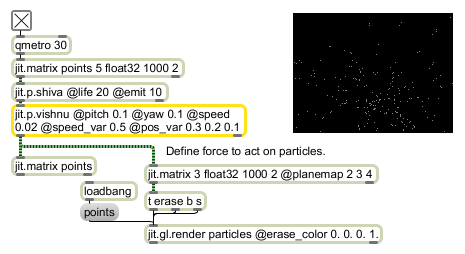# jit.p.vishnu

Apply single force to particles

## Description

The jit.p.vishnu object takes a Jitter particle systems matrix as input, and applies a specific single force world model to it. In order to do so, it interprets the matrix's planes in a particular way: if the incoming matrix is 5-plane, planes 2-4 are interpreted as 3-dimensional position data (x, y, z); if the incoming matrix is 8-plane, planes 2-4 are considered position data, and planes 5-7 as velocity data (in the 5-plane model, velocity is derived from an analysis of positional change).

The world of the jit.p.vishnu object is fairly simple: particles, born in the jit.p.shiva object are animated by applying force to them. New particles (i.e., particles with identical values in both rows 0 and 1) are given an initial position with the pos attribute. Next they are assigned an initial velocity, through the pitch, yaw and speed attributes. Finally, each time the particle passes through the object, the velocity is altered by the world's force.

## Matrix Operator

matrix inputs:1, matrix outputs:1
 Name IOProc Planelink Typelink Dimlink Plane Dim Type out n/a 1 1 1 2 2 float32 float64

## Attributes

Name Type g/s Description
force float The world force per-plane (default = 0. -0.001 0.)
mode int Generation mode flag (default = 0 (use pos attribute))
When the flag is set, any pre-existing values in the matrix are used as initial positions. Otherwise, the pos attribute is used to generate an initial position for new particles.
pitch float The initial angle of deviation from the horizontal plane (in radians) (default = 1.5708)
pitch_var float The amount of variance in pitch attribute output values (default = 0.6981)
pos float The initial position, per-plane (default = 0. -0.5 -0.5)
pos_var float The amount of variance in pos attribute output values (default = 0. 0. 0.)
speed float The initial speed (default = 0.05)
speed_var float The amount of variance in speed attribute output values (default = 0.01)
yaw float The initial angle of deviation from the vertical plane (in radians) (default = 0.)
yaw_var float The amount of variance in yaw attribute output values (default = 6.2832)

## Examples